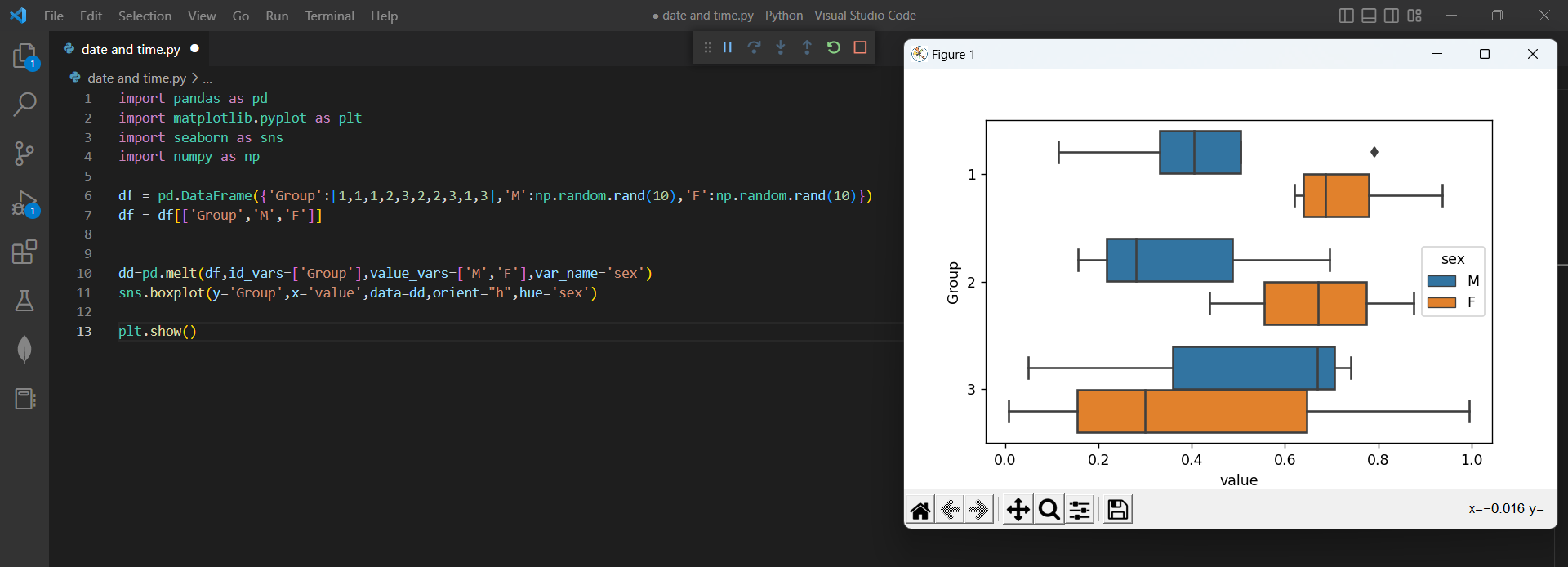# How to Create Boxplots by Group in Pandasby vigneshchennai74Updated: Feb 7, 2023Solution Kit

One of the most popular system for visualizing numerical data in pandas is the boxplot. which can be created by calculating the quartiles of a data set. Box plots are among the most habituated types of graphs in business, statistics, and data analysis.

One way to plot a boxplot using the panda's data frame is to use the boxplot() function that's part of the panda's library. Boxplot is also used to discover the outlier in a data set. Pandas is a Python library built to streamline processes around acquiring and manipulating relational data that has built in methods for plotting and visualizing the values captured in its data structures. The plot() function is used to draw points in a diagram. The plot() function default draws a line from point to point. The function makes parameters for a particular point in the diagram

Box plots are mostly used to show distributions of numeric data values, especially when you want to compare them between multiple groups. These plots are also broadly used for comparing two data sets.

Here is an example of how we can create a boxplot of Grouped columnPreview of the output that you will get on running this code from your IDE

### Code

In this solution we use the boxplot of python

1. Copy the code using the "Copy" button above, and paste it in a Python file in your IDE.
2. Create your own Dataframe that need to be boxploted
4. Run the file to get the Output
5. Add plt.show() at the end of the code to Display the output

I hope you found this useful. I have added the link to dependent libraries, version information in the following sections.

I found this code snippet by searching for "Plotting boxplots for a groupby object" in kandi. You can try any such use case!

Note

• In line 3 make sure the Import sentence starts with small I
• create your own Dataframe for example

df = pd.DataFrame({'Group':[1,1,1,2,3,2,2,3,1,3],'M':np.random.rand(10),'F':np.random.rand(10)})

df = df[['Group','M','F']]

### Environment Tested

I tested this solution in the following versions. Be mindful of changes when working with other versions.

1. The solution is created in Python 3.7.15. Version
2. The solution is tested on numPy 1.21.6 Version
3. The solution is tested on matplotlib 3.5.3 Version
4. The solution is tested on Seaborn 0.12.2 Version

Using this solution, we can able to create boxplot of grouped column using python with the help of pandas library. This process also facilities an easy to use, hassle free method to create a hands-on working version of code which would help us create boxplot in python.

### Dependent Library

pandasby pandas-dev

Python37363Version:v2.0.0rc1Flexible and powerful data analysis / manipulation library for Python, providing labeled data structures similar to R data.frame objects, statistical functions, and much more

Support
Quality
Security
Reuse

pandasby pandas-dev

Python37363Version:v2.0.0rc1License: Permissive (BSD-3-Clause)

Flexible and powerful data analysis / manipulation library for Python, providing labeled data structures similar to R data.frame objects, statistical functions, and much more
Support
Quality
Security
Reuse

numpyby numpy

Python22989Version:v1.24.2The fundamental package for scientific computing with Python.

Support
Quality
Security
Reuse

numpyby numpy

Python22989Version:v1.24.2License: Permissive (BSD-3-Clause)

The fundamental package for scientific computing with Python.
Support
Quality
Security
Reuse

matplotlibby matplotlib

Python17007Version:v3.7.1License: No License (null)

matplotlib: plotting with Python

Support
Quality
Security
Reuse

matplotlibby matplotlib

Python17007Version:v3.7.1License: No License

matplotlib: plotting with Python
Support
Quality
Security
Reuse

Python10488Version:v0.12.2Statistical data visualization in Python

Support
Quality
Security
Reuse

Python10488Version:v0.12.2License: Permissive (BSD-3-Clause)

Statistical data visualization in Python
Support
Quality
Security